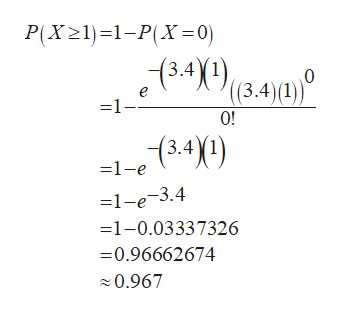Inclusions are defects in poured metal caused by contaminants. The number of (large) inclusions in cast iron follows a Poisson distribution with a rate of 3.4 per cubic millimetre. What is the probability of at least one inclusion in a cubic millimetre? Please enter the answer to 3 decimal places.

Question

Inclusions are defects in poured metal caused by contaminants. The number of (large) inclusions in cast iron follows a Poisson distribution with a rate of 3.4 per cubic millimetre. What is the probability of at least one inclusion in a cubic millimetre? Please enter the answer to 3 decimal places.

Step 1

According to the question, the inclusions follow Poisson distribution which is given by:

Step 2

Where T is the volume of material to inspect,

Now, it is given that the number of inclusions in cast iron follows a Poisson distribution with a rate of 3.4 per cubic millimetre.

So, here

Step 3

Therefore, the probability of at least one inclusi...help_outlineImage TranscriptioncloseP(X21)1-PX=0) 13.41)(3,4)) e 1- 0! (3.4\1) -1-e =1-e-3.4 -1-0.03337326 -0.96662674 0.967 fullscreen

Want to see the full answer?

See Solution

Want to see this answer and more?

Our solutions are written by experts, many with advanced degrees, and available 24/7

See Solution
Tagged in

Probability